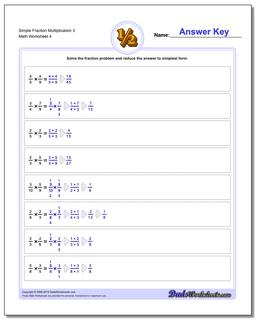# Math Worksheets: Fraction Multiplication: Fraction Multiplication: Simple Fraction Multiplication 3 (Fourth Worksheet)## Simple Fraction Multiplication 3 (Fourth Worksheet)

PropertyValue
DescriptionSimple Fraction Multiplication 3: Basic fraction multiplication, no whole parts or cross-cancelling (Fourth Worksheet)
Resource TypeWorksheet